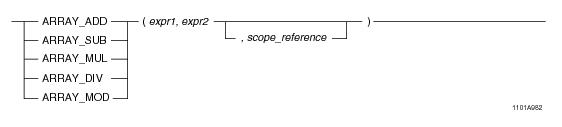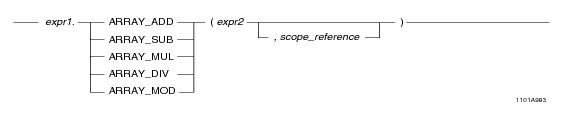# ARRAY Arithmetic Functions - Teradata Database

## SQL Functions, Operators, Expressions, and Predicates

Product
Release Number
15.00
Language
English (United States)
Last Update
2018-09-24
dita:id
B035-1145
lifecycle
previous
Product Category

## ARRAY Arithmetic Functions

### Purpose

Performs arithmetic operations (add, subtract, multiply, divide, and mod) on an ARRAY value, whether referred to in its entirety or over a scope reference.

### Syntax

System function syntax:Method-style syntax:where:

 Syntax element… Specifies… expr1 an ARRAY expression. The element type of the array must be a numeric data type. An ARRAY expression is one of the following: A reference to an ARRAY column An ARRAY constructor expression. See “ARRAY Constructor Expression” on page 195. A UDF expression A UDM expression expr2 an ARRAY expression or a numeric value. The element type of the array must be a numeric data type. An ARRAY expression is one of the following: A reference to an ARRAY column An ARRAY constructor expression. See “ARRAY Constructor Expression” on page 195. A UDF expression A UDM expression scope_reference an optional ARRAY scope reference. See “ARRAY Scope Reference” on page 198.

### ANSI Compliance

This is a Teradata extension to the ANSI SQL:2011 standard.

### Argument Types

Valid arguments for the ARRAY arithmetic functions are one of the following:

• expr1 and expr2 are each an instance of the same ARRAY type.
• expr1 is an ARRAY value with a numeric argument type and expr2 is a numeric value that can be assigned to the element type of expr1.
• If either expr1 or expr2 is NULL, the function returns NULL.

### Usage Notes

For the arithmetic functions ARRAY_ADD, ARRAY_SUB, ARRAY_MUL, ARRAY_DIV, and ARRAY_MOD, two forms are supported for ARRAY types.

In the first form, both left-hand side (LHS) and right-hand side (RHS) operands are two instances of the same ARRAY type.

The arithmetic operation is performed in a pairwise fashion, one by one for each matching pair of elements in the two arguments. If the optional argument scope_reference is defined, then the operation is performed only over the elements within the scope. All elements outside the boundaries of scope_reference are set to NULL. The result is an ARRAY of the same data type as the input ARRAY arguments, with cardinality (number of populated elements) the same as the first input ARRAY argument.

In the second form, one of either LHS or RHS is an ARRAY value with a numeric argument type, and RHS is a numeric value that can be assigned to the element type of LHS. The arithmetic operation is performed by applying the RHS value with the arithmetic operation to each element of the ARRAY argument on the LHS. The result is an ARRAY of the same type as the input ARRAY argument, with cardinality (number of populated elements) the same as the input ARRAY argument. If the optional argument scope_reference is defined, then the operation is performed only over the elements within the scope. All elements outside the boundaries of scope_reference are set to NULL.

For both forms of the arithmetic functions, if a NULL element is encountered in an ARRAY argument, the resulting ARRAY will contain a NULL element in that position. Arithmetic operations involving arrays are not affected by the optional DEFAULT NULL clause in the CREATE TYPE statement. However, the probability of getting an error is increased when comparing arrays that have been created without the DEFAULT NULL clause.

If an ARRAY argument contains any elements that are in an uninitialized state, an error is returned. Use a scope reference to avoid referencing a range of the ARRAY that contains uninitialized elements, or set any uninitialized elements to NULL. You can do this with the OEXTEND method. See “OEXTEND” on page 272.

### Result Type and Value

The result is an ARRAY of the same size as the maximum cardinality of the input array arguments, and the element type is the same as that of expr1. Note that the size of the result array type refers to its current cardinality.

### Example

Consider the following 1-D ARRAY data type and table.

`CREATE TYPE item_price AS DECIMAL(7,2) ARRAY;`
` `
`CREATE TABLE inventory (itemkind INTEGER, `
`                        regular_price item_price,`
`                        sale_price_diff item_price);`

Assume the following element values for the sale_price_diff and regular_price arrays:

`sale_price_diff[1:20] =  100, 200, 120, 140, 50, 160, 45, 10, 90, 100`
`                         50, 100, 200, 90, 250, 550, 200, 200, 50, 75`
`regular_price[1:20] =  50, 90, 80, 10, 45, 30, 20, 10, 90, 100, `
`                       100, 300, 230, 110, 500, 550, 200, 400, 100, 150`

The following query returns a 1-D ARRAY of element type item_price. During evaluation, each element within the specified scope in the regular_price array is combined with the corresponding element of the sale_price_diff array using the arithmetic function ARRAY_SUB.

`SELECT ARRAY_SUB(regular_price, sale_price_diff, 10, 20) `
`FROM inventory;`

The query returns a 1-D ARRAY with the following values:

`output_array[1:20] = [ NULL, NULL, NULL, NULL, NULL, NULL, NULL, NULL, NULL, NULL, 50, 200, 30, 20, 250, 0, 0, 200, 50, 75]`

In the following query, a literal value of 2 is added to all elements in the 1-D ARRAY sale_price_diff.

`SELECT ARRAY_ADD(sale_price_diff, 2) FROM inventory;`

This query returns a 1-D ARRAY with the following values:

`output_array[1:20] = [ 102, 202, 122, 142, 52, 162, 47, 12, 92, 102`
`                       52, 102, 202, 92, 252, 552, 202, 202, 52, 77]`

The following query shows the use of a filtering condition while performing arithmetic operations on an ARRAY. In this example, all elements within the scope [10:20] that have a value less than 1000 are multiplied by 2.

`SELECT ARRAY_MUL(regular_price, 2, 10, 20) FROM inventory`
`WHERE ARRAY_COUNT_DISTINCT(ARRAY_LT(regular_price,1000,10,20),1)>1;`

This query returns a 1-D ARRAY with the following values:

`output_array[1:20] = [NULL, NULL, NULL, NULL, NULL, NULL, NULL, NULL, NULL, NULL, 200, 600, 460, 220, 1000, 1100, 400, 800, 200, 300]`

### Example

Consider the following 2-D ARRAY data type and table:

`CREATE TYPE shot_ary AS VARRAY(1:50)(1:50) OF INTEGER DEFAULT NULL;`
` `
`CREATE TABLE seismic_data (`
`   id INTEGER,`
`   shot1 shot_ary,`
`   shot2 shot_ary);`

The following query returns an ARRAY of type shot_ary with elements in scope reference [10:20][10:20] modified as a result of the operation. During evaluation, each element within the specified scope reference in shot1 is combined with the corresponding element of shot2 using the arithmetic function ARRAY_ADD.

`SELECT ARRAY_ADD(shot1, shot2, NEW arrayVec(10, 20), `
`   NEW arrayVec(10,20)) `
`FROM seismic_data;`

In the following query, a literal value of 9 is added to all elements within the specified scope reference of the shot1 array.

`SELECT ARRAY_ADD(shot1, 9, NEW arrayVec(10,10), NEW arrayVec(20,20)) FROM seismic_data;`

The following is the same query using method-style syntax:

`SELECT shot1.ARRAY_ADD(9, NEW arrayVec(10,10), NEW arrayVec(20,20)) FROM seismic_data;`

The following query shows the use of a filtering condition while performing arithmetic operations on an n-D ARRAY. In this example, all elements within the scope reference [10:20][10:20] that have a negative value are multiplied by zero.

`SELECT ARRAY_MUL(shot1, 0, NEW arrayVec(10,10), NEW arrayVec(20,20)) FROM seismic_data`
`WHERE ARRAY_COUNT_DISTINCT(ARRAY_LT(shot1, 0, NEW arrayVec(10,10), `
`   NEW arrayVec(20,20)),0)>1;`